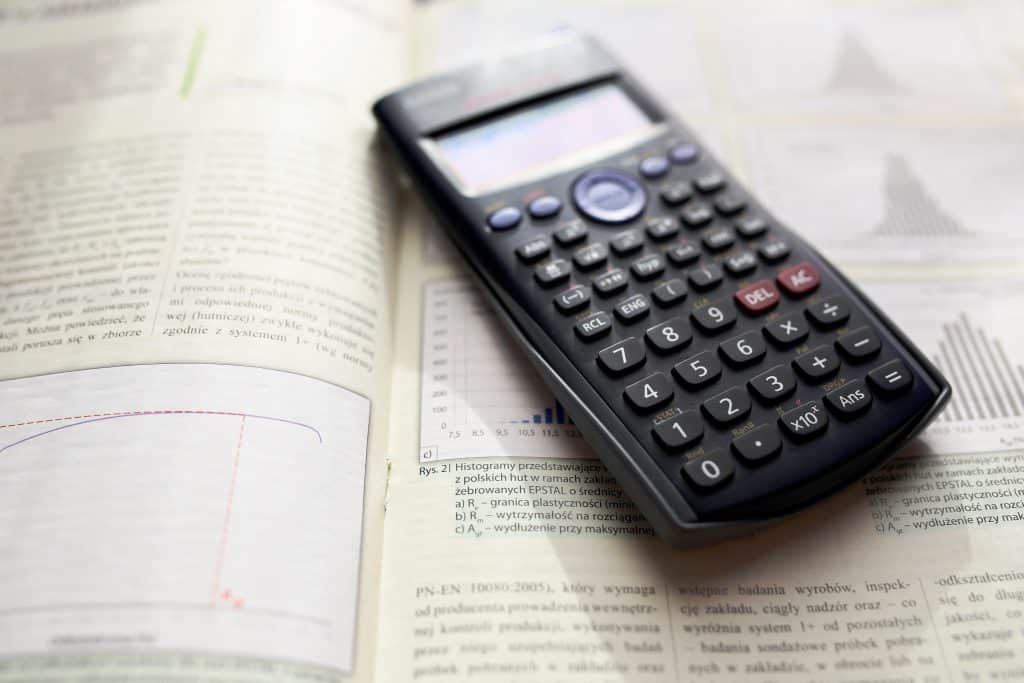# The Sharpe Ratio FormulaThe Sharpe Ratio was developed it in 1966 by William F. Sharpe as a way to quantify potential risk in an individual investment or an investing method or trading strategy.

The Sharpe Ratio is the defined difference of the returns between an investment and the potential risk free return that is then divided by the standard deviation/volatility of the investment as a comparison. The Sharpe Ratio is a representation of the returns above what an investor would receive per unit of the increase in risk.

The Sharpe Ratio quantifies if the return of an investment compensates the investor enough for the amount of risk that is taken in comparison to the potential return. In a comparison of two different investments to a relating benchmark, the asset with the highest Sharpe Ratio provides better risk adjusted returns or similar returns for less risk.

The Sharpe Ratio Formula:

Sharpe Ratio = (Rx – Rf) / StdDev Rx

Where:

• Rx = Expected portfolio return
• Rf = Risk free rate of return
• StdDev Rx = Standard deviation of portfolio return / volatility

A Sharpe ratio less than 1.0 is poor.

A Sharpe ratio of 1.0 is acceptable.

A Sharpe ratio of 2.0 is good.

A Sharpe ratio of 3.0 is excellent.